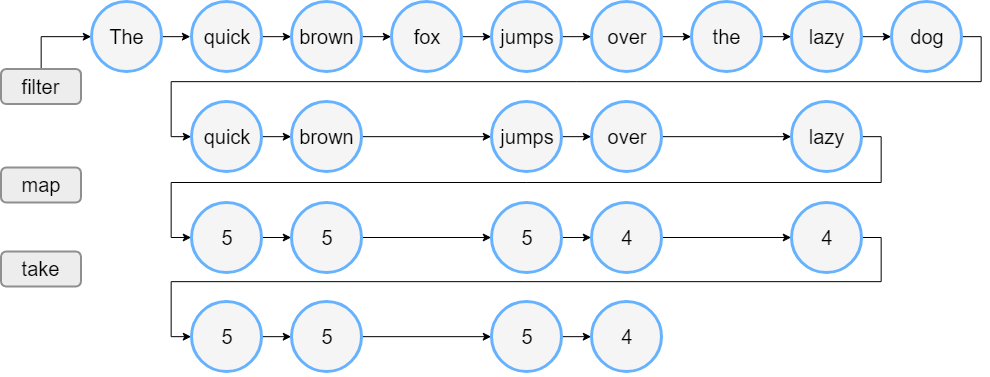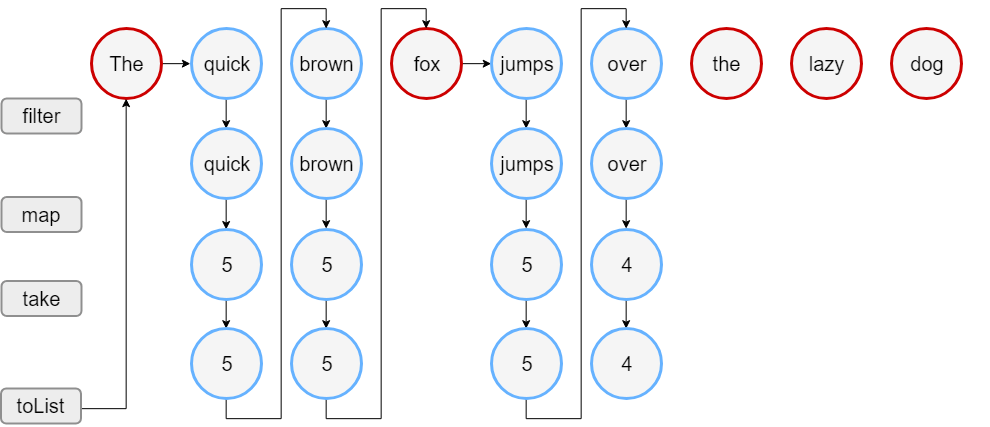/Kotlin

Sequences

Along with collections, the Kotlin standard library contains another container type – sequences (Sequence<T>). Sequences offer the same functions as Iterable but implement another approach to multi-step collection processing.

When the processing of an Iterable includes multiple steps, they are executed eagerly: each processing step completes and returns its result – an intermediate collection. The following step executes on this collection. In turn, multi-step processing of sequences is executed lazily when possible: actual computing happens only when the result of the whole processing chain is requested.

The order of operations execution is different as well: Sequence performs all the processing steps one-by-one for every single element. In turn, Iterable completes each step for the whole collection and then proceeds to the next step.

So, the sequences let you avoid building results of intermediate steps, therefore improving the performance of the whole collection processing chain. However, the lazy nature of sequences adds some overhead which may be significant when processing smaller collections or doing simpler computations. Hence, you should consider both Sequence and Iterable and decide which one is better for your case.

Constructing

From elements

To create a sequence, call the sequenceOf() function listing the elements as its arguments.

val numbersSequence = sequenceOf("four", "three", "two", "one")

From Iterable

If you already have an Iterable object (such as a List or a Set), you can create a sequence from it by calling asSequence().

val numbers = listOf("one", "two", "three", "four")

From function

One more way to create a sequence is by building it with a function that calculates its elements. To build a sequence based on a function, call generateSequence() with this function as an argument. Optionally, you can specify the first element as an explicit value or a result of a function call. The sequence generation stops when the provided function returns null. So, the sequence in the example below is infinite.

fun main() {
//sampleStart
val oddNumbers = generateSequence(1) { it + 2 } // `it` is the previous element
println(oddNumbers.take(5).toList())
//println(oddNumbers.count())     // error: the sequence is infinite
//sampleEnd
}

To create a finite sequence with generateSequence(), provide a function that returns null after the last element you need.

fun main() {
//sampleStart
val oddNumbersLessThan10 = generateSequence(1) { if (it + 2 < 10) it + 2 else null }
println(oddNumbersLessThan10.count())
//sampleEnd
}

From chunks

Finally, there is a function that lets you produce sequence elements one by one or by chunks of arbitrary sizes – the sequence() function. This function takes a lambda expression containing calls of yield() and yieldAll() functions. They return an element to the sequence consumer and suspend the execution of sequence() until the next element is requested by the consumer. yield() takes a single element as an argument; yieldAll() can take an Iterable object, an Iterator, or another Sequence. A Sequence argument of yieldAll() can be infinite. However, such a call must be the last: all subsequent calls will never be executed.

fun main() {
//sampleStart
val oddNumbers = sequence {
yield(1)
yieldAll(listOf(3, 5))
yieldAll(generateSequence(7) { it + 2 })
}
println(oddNumbers.take(5).toList())
//sampleEnd
}

Sequence operations

The sequence operations can be classified into the following groups regarding their state requirements:

• Stateless operations require no state and process each element independently, for example, map() or filter(). Stateless operations can also require a small constant amount of state to process an element, for example, take() or drop().
• Stateful operations require a significant amount of state, usually proportional to the number of elements in a sequence.

If a sequence operation returns another sequence, which is produced lazily, it's called intermediate. Otherwise, the operation is terminal. Examples of terminal operations are toList() or sum(). Sequence elements can be retrieved only with terminal operations.

Sequences can be iterated multiple times; however some sequence implementations might constrain themselves to be iterated only once. That is mentioned specifically in their documentation.

Sequence processing example

Let's take a look at the difference between Iterable and Sequence with an example.

Iterable

Assume that you have a list of words. The code below filters the words longer than three characters and prints the lengths of first four such words.

fun main() {
//sampleStart
val words = "The quick brown fox jumps over the lazy dog".split(" ")
val lengthsList = words.filter { println("filter: \$it"); it.length > 3 }
.map { println("length: \${it.length}"); it.length }
.take(4)

println("Lengths of first 4 words longer than 3 chars:")
println(lengthsList)
//sampleEnd
}

When you run this code, you'll see that the filter() and map() functions are executed in the same order as they appear in the code. First, you see filter: for all elements, then length: for the elements left after filtering, and then the output of the two last lines. This is how the list processing goes:Sequence

Now let's write the same with sequences:

fun main() {
//sampleStart
val words = "The quick brown fox jumps over the lazy dog".split(" ")
//convert the List to a Sequence
val wordsSequence = words.asSequence()

val lengthsSequence = wordsSequence.filter { println("filter: \$it"); it.length > 3 }
.map { println("length: \${it.length}"); it.length }
.take(4)

println("Lengths of first 4 words longer than 3 chars")
// terminal operation: obtaining the result as a List
println(lengthsSequence.toList())
//sampleEnd
}

The output of this code shows that the filter() and map() functions are called only when building the result list. So, you first see the line of text “Lengths of..” and then the sequence processing starts. Note that for elements left after filtering, the map executes before filtering the next element. When the result size reaches 4, the processing stops because it's the largest possible size that take(4) can return.

The sequence processing goes like this:In this example, the sequence processing takes 18 steps instead of 23 steps for doing the same with lists.

© 2010–2020 JetBrains s.r.o. and Kotlin Programming Language contributors